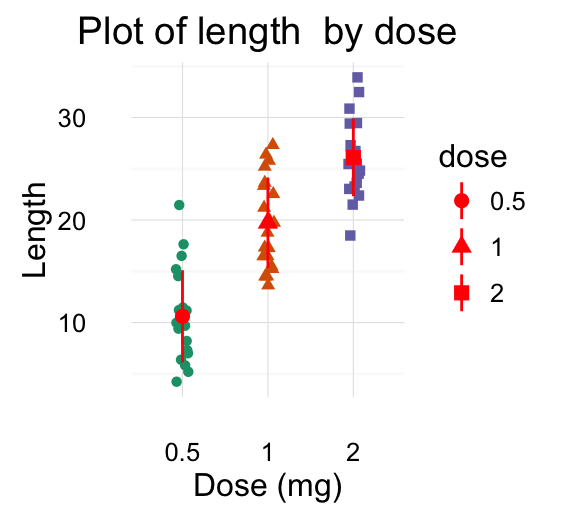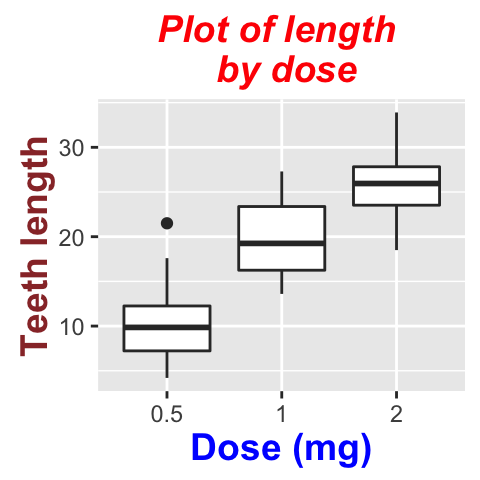Introduction

ggplot2 is a powerful and a flexible R package, implemented by Hadley Wickham, for producing elegant graphics.

The concept behind ggplot2 divides plot into three different fundamental parts: Plot = data + Aesthetics + Geometry.

The principal components of every plot can be defined as follow:

• data is a data frame
• Aesthetics is used to indicate x and y variables. It can also be used to control the color, the size or the shape of points, the height of bars, etc…..
• Geometry defines the type of graphics (histogram, box plot, line plot, density plot, dot plot, ….)

There are two major functions in ggplot2 package: qplot() and ggplot() functions.

• qplot() stands for quick plot, which can be used to produce easily simple plots.
• ggplot() function is more flexible and robust than qplot for building a plot piece by piece.

This document provides R course material for producing different types of plots using ggplot2.

If you want be highly effective, download our book: Guide to Create Beautiful Graphics in R# Installation
install.packages('ggplot2')
library(ggplot2)

Data format and preparation

The data should be a data.frame (columns are variables and rows are observations).

The data set mtcars is used in the examples below:

data(mtcars)
df <- mtcars[, c("mpg", "cyl", "wt")]
##                    mpg cyl    wt
## Mazda RX4         21.0   6 2.620
## Mazda RX4 Wag     21.0   6 2.875
## Datsun 710        22.8   4 2.320
## Hornet 4 Drive    21.4   6 3.215
## Hornet Sportabout 18.7   8 3.440
## Valiant           18.1   6 3.460

Plotting with ggplot2

1. qplot(): Quick plot with ggplot2
• Scatter plots
• Bar plot
• Box plot, violin plot and dot plot
• Histogram and density plots
2. Box plots
• Basic box plots
• Box plot with dots
• Change box plot colors by groups
• Change box plot line colors
• Change box plot fill colors
• Change the legend position
• Change the order of items in the legend
• Box plot with multiple groups
• Functions: geom_boxplot(), stat_boxplot(), stat_summary()1. Violin plots
• Basic violin plots
• Add summary statistics on a violin plot
• Add mean and median points
• Add mean and standard deviation
• Violin plot with dots
• Change violin plot colors by groups
• Change violin plot line colors
• Change violin plot fill colors
• Change the legend position
• Change the order of items in the legend
• Violin plot with multiple groups
• Functions: geom_violin(), stat_ydensity()1. Dot plots
• Basic dot plots
• Add summary statistics on a dot plot
• Add mean and median points
• Dot plot with box plot and violin plot
• Add mean and standard deviation
• Change dot plot colors by groups
• Change the legend position
• Change the order of items in the legend
• Dot plot with multiple groups
• Functions: geom_dotplot()1. Stripcharts
• Basic stripcharts
• Add summary statistics on a stripchart
• Add mean and median points
• Stripchart with box blot and violin plot
• Add mean and standard deviation
• Change point shapes by groups
• Change stripchart colors by groups
• Change the legend position
• Change the order of items in the legend
• Stripchart with multiple groups
• Functions: geom_jitter(), stat_summary()1. Density plots
• Basic density plots
• Change density plot line types and colors
• Change density plot colors by groups
• Calculate the mean of each group :
• Change line colors
• Change fill colors
• Change the legend position
• Combine histogram and density plots
• Use facets
• Functions: geom_density(), stat_density()1. Histogram plots
• Basic histogram plots
• Add mean line and density plot on the histogram
• Change histogram plot line types and colors
• Change histogram plot colors by groups
• Calculate the mean of each group
• Change line colors
• Change fill colors
• Change the legend position
• Use facets
• Functions: geom_histogram(), stat_bin(), position_identity(), position_stack(), position_dodge().1. Scatter plots
• Basic scatter plots
• Label points in the scatter plot
• Change the appearance of points and lines
• Scatter plots with multiple groups
• Change the point color/shape/size automatically
• Change the point color/shape/size manually
• Add marginal rugs to a scatter plot
• Scatter plots with the 2d density estimation
• Scatter plots with ellipses
• Scatter plots with rectangular bins
• Scatter plot with marginal density distribution plot
• Functions: geom_point(), geom_smooth(), stat_smooth(), geom_rug(), geom_density_2d(), stat_density_2d(), stat_bin_2d(), geom_bin2d(), stat_summary_2d(), geom_hex() (see stat_bin_hex()), stat_summary_hex()1. Bar plots
• Basic bar plots
• Bar plot with labels
• Bar plot of counts
• Change bar plot colors by groups
• Change outline colors
• Change fill colors
• Change the legend position
• Change the order of items in the legend
• Bar plot with multiple groups
• Bar plot with a numeric x-axis
• Bar plot with error bars
• Functions: geom_bar(), geom_errorbar()1. Line plots
• Line types in R
• Basic line plots
• Line plot with multiple groups
• Change globally the appearance of lines
• Change automatically the line types by groups
• Change manually the appearance of lines
• Functions: geom_line(), geom_step(), geom_path(), geom_errorbar()1. Error bars
• Add error bars to a bar and line plots
• Bar plot with error bars
• Line plot with error bars
• Dot plot with mean point and error bars
• Functions: geom_errorbarh(), geom_errorbar(), geom_linerange(), geom_pointrange(), geom_crossbar(), stat_summary()
2. Pie chart
• Simple pie charts
• Change the pie chart fill colors
• Create a pie chart from a factor variable
• Functions: coord_polar()1. QQ plots
• Basic qq plots
• Change qq plot point shapes by groups
• Change qq plot colors by groups
• Change the legend position
• Functions: stat_qq()1. ggsave(): Save a ggplot
• print(): print a ggplot to a file
• ggsave: save the last ggplot
• Functions: print(), ggsave()

Graphical parameters

1. Main title, axis labels and legend title
• Change the main title and axis labels
• Change the appearance of the main title and axis labels
• Remove x and y axis labels
• Functions: labs(), ggtitle(), xlab(), ylab(), update_labels()1. Legend position and appearance
• Change the legend position
• Change the legend title and text font styles
• Change the background color of the legend box
• Change the order of legend items
• Remove the plot legend
• Remove slashes in the legend of a bar plot
• guides() : set or remove the legend for a specific aesthetic
• Functions: guides(), guide_legend(), guide_colourbar()1. Change colors automatically and manually
• Use a single color
• Change colors by groups
• Default colors
• Change colors manually
• Use RColorBrewer palettes
• Use Wes Anderson color palettes
• Use gray colors
• Functions:
• Brewer palettes: scale_colour_brewer(), scale_fill_brewer(), scale_color_brewer()
• Gray scales: scale_color_grey(), scale_fill_grey()
• Manual colors: scale_color_manual(), scale_fill_manual()
• Hue colors: scale_colour_hue()1. Point shapes, colors and size
• Change the point shapes, colors and sizes automatically
• Change point shapes, colors and sizes manually
• Functions: scale_shape_manual(), scale_color_manual(), scale_size_manual()

Points shapes available in R:1. Add text annotations to a graph
• Text annotations using the function geom_text
• Change the text color and size by groups
• Add a text annotation at a particular coordinate
• annotation_custom : Add a static text annotation in the top-right, top-left, …
• Functions: geom_text(), annotate(), annotation_custom()1. Line types
• Line types in R
• Basic line plots
• Line plot with multiple groups
• Change globally the appearance of lines
• Change automatically the line types by groups
• Change manually the appearance of lines
• Functions: scale_linetype(), scale_linetype_manual(), scale_color_manual(), scale_size_manual()1. Themes and background colors
• Quick functions to change plot themes
• Customize the appearance of the plot background
• Change the colors of the plot panel background and the grid lines
• Remove plot panel borders and grid lines
• Change the plot background color (not the panel)
• Use a custom theme
• theme_tufte : a minimalist theme
• theme_economist : theme based on the plots in the economist magazine
• theme_stata: theme based on Stata graph schemes.
• theme_wsj: theme based on plots in the Wall Street Journal
• theme_calc : theme based on LibreOffice Calc
• theme_hc : theme based on Highcharts JS
• Functions: theme(), theme_bw(), theme_grey(), theme_update(), theme_blank(), theme_classic(), theme_minimal(), theme_void(), theme_dark(), element_blank(), element_line(), element_rect(), element_text(), rel()1. Axis scales and transformations
• Change x and y axis limits
• Use xlim() and ylim() functions
• Use expand_limts() function
• Use scale_xx() functions
• Axis transformations
• Log and sqrt transformations
• Format axis tick mark labels
• Display log tick marks
• Format date axes
• Plot with dates
• Format axis tick mark labels
• Date axis limits
• Functions:
• xlim(), ylim(), expand_limits() : x, y axis limits
• scale_x_continuous(), scale_y_continuous()
• scale_x_log10(), scale_y_log10(): log10 transformation
• scale_x_sqrt(), scale_y_sqrt(): sqrt transformation
• coord_trans()
• scale_x_reverse(), scale_y_reverse()
• annotation_logticks()
• scale_x_date(), scale_y_date()
• scale_x_datetime(), scale_y_datetime()1. Axis ticks: customize tick marks and labels, reorder and select items
• Change the appearance of the axis tick mark labels
• Hide x and y axis tick mark labels
• Change axis lines
• Set axis ticks for discrete and continuous axes
• Customize a discrete axis
• Change the order of items
• Change tick mark labels
• Choose which items to display
• Customize a continuous axis
• Set the position of tick marks
• Format the text of tick mark labels
• Functions: theme(), scale_x_discrete(), scale_y_discrete(), scale_x_continuous(), scale_y_continuous()1. Add straight lines to a plot: horizontal, vertical and regression lines
• geom_hline : Add horizontal lines
• geom_vline : Add vertical lines
• geom_abline : Add regression lines
• geom_segment : Add a line segment
• Functions: geom_hline(), geom_vline(), geom_abline(), geom_segment()1. Rotate a plot: flip and reverse
• Horizontal plot : coord_flip()
• Reverse y axis
• Functions: coord_flip(), scale_x_reverse(), scale_y_reverse()1. Faceting: split a plot into a matrix of panels
• Facet with one variable
• Facet with two variables
• Facet scales
• Facet labels
• facet_wrap
• Functions: facet_grid(), facet_wrap(), label_both(), label_bquote(), label_parsed()Infos

This analysis was performed using R (ver. 3.2.4) and ggplot2 (ver 2.1.0).